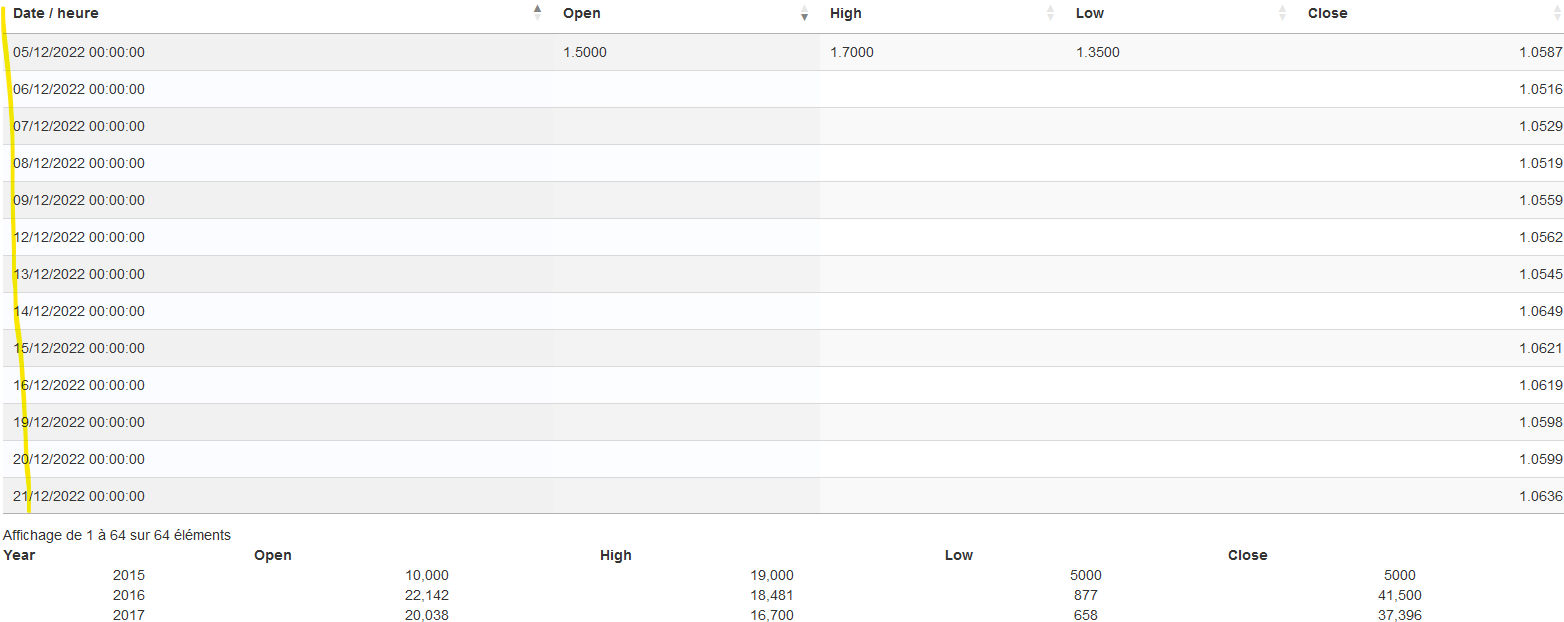# Datatables and Highcharts - table.on('draw' and function chartData(table)

Hello,
I managed to buid a Highcharts more or less the way I wanted based on a table that is similar to what I can get through Datatables, see working example. Unfortunately, when I link the Highcharts to my Datatables, the Highcharts is blank, probably beccause I am missing the

``````table.on('draw', function () {
});
``````

and the

``````function chartData(table) {
}
``````

parts of my js, as described here or here.

Would you know how I could write these parts to fit my case? I don't need to count, sum or average the data, but just read the value in the Datatables.

• probably beccause I am missing the [...] parts

Given that those are the parts that populate the chart, you are spot onYou would use the `rows().data()` method to get the data from the table, and then plot that into Highcharts using their API. Perhaps you can show us what your DataTable looks like?

Allan

• Hi Allan,

My datatable is highlighted in yellow. It contains the same columns as the table of my working example.• That's one axis (presumably horizontal). Is the data in the 5th column the one you want to plot it against? If so:

``````var myTable = new DataTable.Api('#myTable'); // or \$('#myTable').DataTable();, `table` if you already have it, etc

var xData = myTable.column(0).data().toArray();
var yData = myTable.column(4).data().toArray();
``````

That uses `column().data()` to get the data for the column selected.

Allan

• Hi Allan,

I am sorry but I don't know how to use this information.
Here is my code

``````    var table = \$('#quotes').DataTable( {
ajax: 'php/table.quotes.php',
columns: [
{"data": "currency_rate.quote_datetime"},
{"data": "currency_rate.quote_open"},
{"data": "currency_rate.quote_high"},
{"data": "currency_rate.quote_low"},
{"data": "currency_rate.quote_last"}
]
});

//  var xData = quotes.column(0).data().toArray();
//  var yData = quotes.column(4).data().toArray();

var chart = Highcharts.chart("container", {
data: {
table: quotes,
complete: function(options) {
options.series.forEach(series => {
series.data = series.data.map(function(data) {
if (typeof data === 'string') {
return [data, parseInt(data.replace(/,/g, ''))];
} else {
return [data, data];
}
});
});
}
},
chart: {
type: 'line',
displayErrors: true
},
accessibility: {
enabled: true
},
title: {
text: "Test Data"
},
xAxis: {
type: 'category'
},
});

``````
• Here is a simple example based on the HighCharts link you gave originally: https://jsbin.com/dohejepido/1/edit?html%2Cjs%2Coutput= .

I've only done a single series - the rest can readily be added.

Allan

• Hi Allan,
Thank you.
I will work on trying to add some more columns, but this time, when I apply the code to my Datatable, I get the following error message: "Uncaught TypeError: d is undefined".
I don't understand as both tables are very similar.

• Can you update Allan's example to show the issue so we can take a look?

Kevin

• The problem is that I cannot update Allan's example because I get no error message with the table id="example1", which works fine.
The error message comes when linking the Highchart to my "real table".
I will try to give access.

• Yes - we'll need to be able to see the issue to be able to offer any help debugging it.

Allan